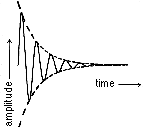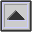DECAY TIME

The time required for the AMPLITUDE of a vibrating system to decrease to approximately 37% (or 1/e) of its initial value. For a system with a constant rate of DAMPING, the DECAY is exponential and the decay time is independent of the initial amplitude.

Compare: ATTACK, RATE OF DECAY, RISE TIME.Exponential damping of a sine wave. Dashed line indicates exponential decay of the amplitude.

home